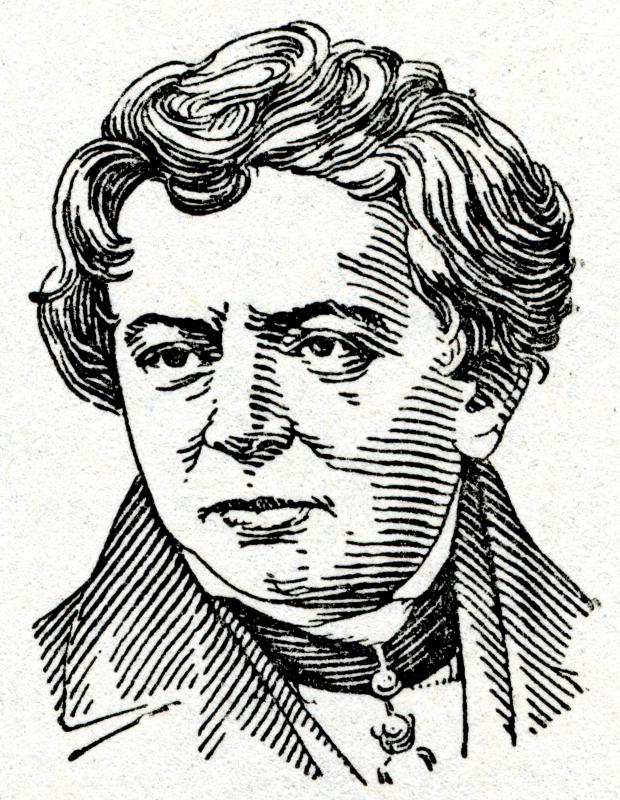# What Is Ohm's Law?

Karize Uy

Ohm’s Law is a law used in physics that basically explains how electricity operates properly within a simple circuit. In order to explain the electrical process, the law shows how the three elements of electricity — ampere, resistance, and voltage — work together to create a functioning electrical circuit. The law states that the amount of electrical current, measured in amperes, traveling through a conductor is proportional or equal to the voltage, but is inversely proportional to the resistance in the conductor.Ohm's Law can be used to find the electrical resistance applied to a circuit by resistors.

The proponent and the namesake of the law was George Simon Ohm, a renowned German physicist in the early 1800s. While working as a professor at the Jesuit Gymnasium of Cologne in Germany, he experimented with and observed the behavior of electricity in simple circuits with different wire lengths. He described and documented all the results in a book, “The Galvanic Circuit Investigated Mathematically," which was initially rejected but later acknowledged, leading to the establishment of the Ohm’s Law.German physicist Georg Ohm uncovered how a material's make up, length and thickness influences how much current will flow through it at a certain voltage.

Ohm’s Law can be written in a simple mathematical equation: I = V/R, where I is for the electrical current measured in amperes, V is for the voltage, and R is for the resistance. In this equation, the resistance is usually a constant variable, since its value is not dependent on the amount of electric current, but rather on the materials used to make the circuit, such as the metal wires and the resistor itself. The formula can be expressed in other inversed forms such as V = IR, or R = V/I. These inversed formulas can help find the value of one element if the values of the two other elements are already identified.

There are essentially three “truth” statements that one should remember regarding Ohm’s Law. The first statement is that the value of I will increase or decrease if the value of V increases or decreases, respectively. The second statement is that the value of I will decrease if the value of R increases and the value of V does not change. The third statement is that the value of I will increase if the value of R decreases and the value of V remains the same.

The principle of Ohm’s Law can be practically applied in appliances and any equipment operated by electricity or a battery. For example, a simple light-emitting diode (LED) needs only 2 volts and .02 amperes to light up, but is connected to a 6-volt battery. This may cause the LED to short circuit, and a resistor is needed to reduce the current. Using the formula R = V/I, one can determine that a resistor containing 200 ohms is needed to control the current coming into the LED.A resistor is an electronic component that can lower a circuit’s voltage and its flow of electrical current.

## You might also Like